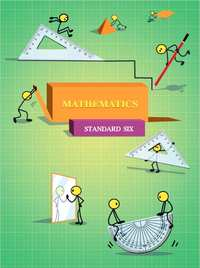# Balbharati solutions for Mathematics 6th Standard Maharashtra State Board chapter 3 - Integers [Latest edition]

#### Chapters## Chapter 3: Integers

Practice Set 4Practice Set 5Practice Set 6Practice Set 7Practice Set 8
Practice Set 4 [Page 15]

### Balbharati solutions for Mathematics 6th Standard Maharashtra State Board Chapter 3 Integers Practice Set 4 [Page 15]

Practice Set 4 | Q 1 | Page 15

Classify the following numbers as positive numbers and negative numbers.
–5, +4, –2, 7, +26, –49, –37, 19, –25, +8, 5, –4, –12, 27

Practice Set 4 | Q 2 | Page 15

Given below are the temperatures in some cities. Write to them using the proper signs.

 Place Shimla Leh Delhi Nagpur Temperature 7°C above 0° 12°C above 0° 22°C above 0° 31°C above 0°
Practice Set 4 | Q 3.1 | Page 15

Write the number in the following example using the proper signs.

A submarine is at a depth of 512 metres below sea level.

Practice Set 4 | Q 3.2 | Page 15

Write the number in the following example using the proper signs.

The height of Mt Everest, the highest peak in the Himalayas, is 8848 metres.

Practice Set 4 | Q 3.3 | Page 15

Write the number in the following example using the proper signs.

A kite is flying at a distance of 120 metres from the ground.

Practice Set 4 | Q 3.4 | Page 15

Write the number in the following example using the proper signs.

The tunnel is at a depth of 2 metres under the ground.

Practice Set 5 [Page 18]

### Balbharati solutions for Mathematics 6th Standard Maharashtra State Board Chapter 3 Integers Practice Set 5 [Page 18]

Practice Set 5 | Q 1.1 | Page 18

Practice Set 5 | Q 1.2 | Page 18

Practice Set 5 | Q 1.3 | Page 18

Practice Set 5 | Q 1.4 | Page 18

Practice Set 5 | Q 1.5 | Page 18

Practice Set 5 | Q 1.6 | Page 18

Practice Set 5 | Q 2 | Page 18

Complete the table given below.

 + 8 4 –3 –5 –2 –2 + 8 = +16 6 0 –4
Practice Set 6 [Page 19]

### Balbharati solutions for Mathematics 6th Standard Maharashtra State Board Chapter 3 Integers Practice Set 6 [Page 19]

Practice Set 6 | Q 1 | Page 19

Write the opposite number of each of the numbers given below.

 Number 47 + 52 – 33 – 84 – 21 + 16 – 26 80 Opposite number
Practice Set 7 [Page 19]

### Balbharati solutions for Mathematics 6th Standard Maharashtra State Board Chapter 3 Integers Practice Set 7 [Page 19]

Practice Set 7 | Q 1 | Page 19

Write the proper sings > , < or = in the boxes below.

 (1) –4 ____ 5 (2) 8 ___ –10 (3) +9 ____ +9 (4) – 6 ___ 0 (5) 7 ______ 4 (6) 3 ___ 0 (7) –7 ___ 7 (8) –12 ____ 5 (9) –2 _____ –8 (10) –1 __  –2 (11) 6 ___ −3 (12) –14 ____  –14
Practice Set 8 [Page 20]

### Balbharati solutions for Mathematics 6th Standard Maharashtra State Board Chapter 3 Integers Practice Set 8 [Page 20]

Practice Set 8 | Q 1 | Page 20

Subtract the numbers in the top row from the numbers in the first column and write the proper number in each empty box.

 – 6 9 –4 –5 0 +7 –8 –3 3 3 – 6 = –3 8 8 − (−5) = 13 –3 –2

## Chapter 3: Integers

Practice Set 4Practice Set 5Practice Set 6Practice Set 7Practice Set 8## Balbharati solutions for Mathematics 6th Standard Maharashtra State Board chapter 3 - Integers

Balbharati solutions for Mathematics 6th Standard Maharashtra State Board chapter 3 (Integers) include all questions with solution and detail explanation. This will clear students doubts about any question and improve application skills while preparing for board exams. The detailed, step-by-step solutions will help you understand the concepts better and clear your confusions, if any. Shaalaa.com has the Maharashtra State Board Mathematics 6th Standard Maharashtra State Board solutions in a manner that help students grasp basic concepts better and faster.

Further, we at Shaalaa.com provide such solutions so that students can prepare for written exams. Balbharati textbook solutions can be a core help for self-study and acts as a perfect self-help guidance for students.

Concepts covered in Mathematics 6th Standard Maharashtra State Board chapter 3 Integers are Concept of Integers, Concept for Natural Numbers, Concept for Whole Numbers, Negative and Positive Numbers, Representation of Integers on the Number Line, Concept for Ordering of Integers, Additive Inverse, Subtraction of Integers, Addition of Integers, Addition of Integers on Number line.

Using Balbharati 6th Standard solutions Integers exercise by students are an easy way to prepare for the exams, as they involve solutions arranged chapter-wise also page wise. The questions involved in Balbharati Solutions are important questions that can be asked in the final exam. Maximum students of Maharashtra State Board 6th Standard prefer Balbharati Textbook Solutions to score more in exam.

Get the free view of chapter 3 Integers 6th Standard extra questions for Mathematics 6th Standard Maharashtra State Board and can use Shaalaa.com to keep it handy for your exam preparation